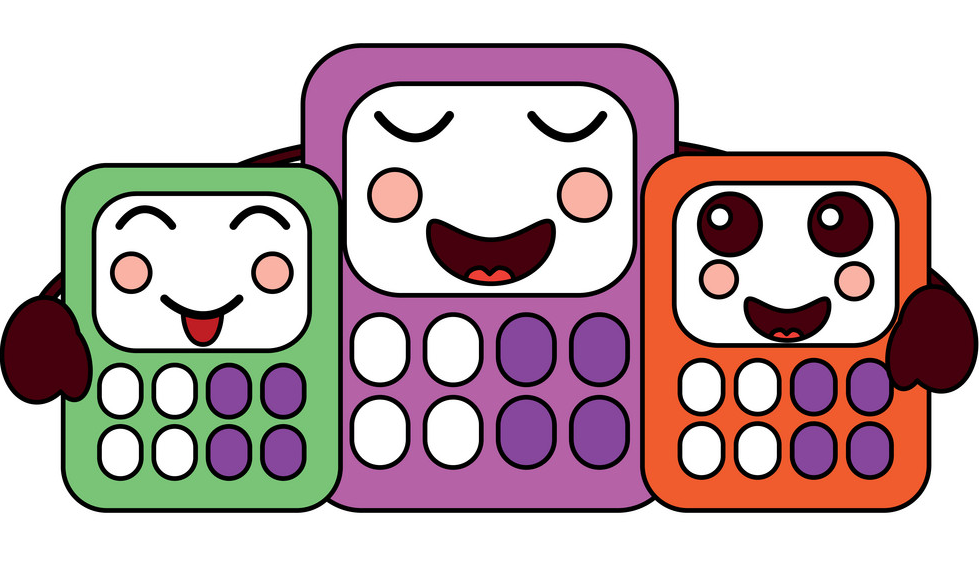# CALCULATORSA calculator is a device that performs arithmetic operations on numbers. The simplest calculators can do only addition, subtraction, multiplication, and division. More sophisticated calculators can handle exponent dial operations, roots, logarithm s, trigonometric functions, and hyperbolic functions.

(Source : whatis.techtarget.com)